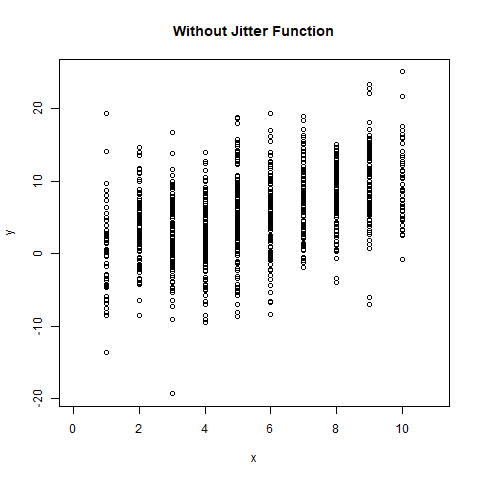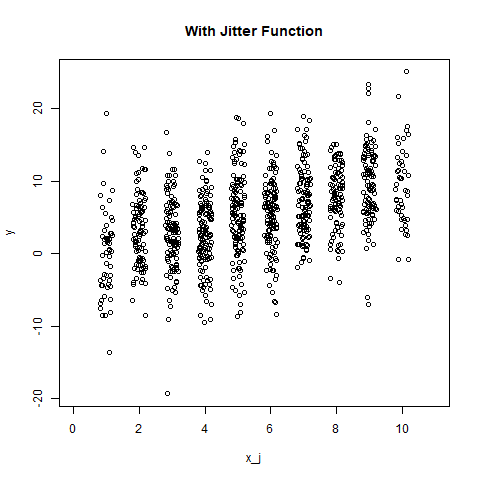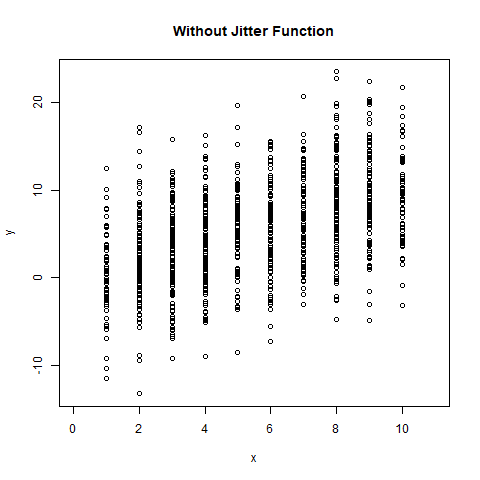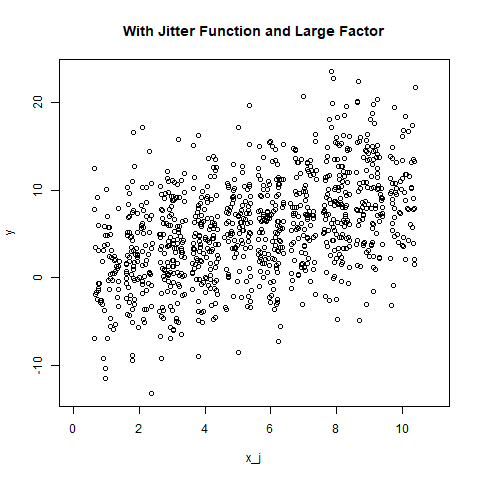# Adding Noise to a Numeric Vector in R Programming – jitter() Function

• Last Updated : 19 Jun, 2020

In R programming, jittering means adding small amount of random noise to a numeric vector object. In this article, we’ll learn to use `jitter()` function and create a plot to visualize them.

Syntax: jitter(x, factor)

Parameters:
x: represents numeric vector
factor: represents numeric value for factor specification

Example 1:

 `# Define numeric vectors``x <- ``round``(``runif``(1000, 1, 10))``y <- x + ``rnorm``(1000, mean = 0, sd = 5)`` ` `# output to be present as PNG file ``png``(file=``"withoutJitter.png"``)`` ` `# Plotting without jitter function``plot``(x, y, xlim = ``c``(0, 11),``     ``main = ``"Without Jitter Function"``)`` ` `# saving the file ``dev.off``()`` ` `x_j <- ``jitter``(x)`` ` `# output to be present as PNG file ``png``(file=``"withJitter.png"``)`` ` `# Plotting with jitter function``plot``(x_j, y, xlim = ``c``(0, 11),``     ``main = ``"With Jitter Function"``)`` ` `# saving the file ``dev.off``()`

Output:Example 2: With large factor value

 `# Define numeric vectors``x <- ``round``(``runif``(1000, 1, 10))``y <- x + ``rnorm``(1000, mean = 0, sd = 5)`` ` `# output to be present as PNG file ``png``(file=``"withoutJitterFactor.png"``)`` ` `# Plotting without jitter function``plot``(x, y, xlim = ``c``(0, 11),``     ``main = ``"Without Jitter Function"``)`` ` `# saving the file ``dev.off``()`` ` `x_j <- ``jitter``(x, factor = 2)`` ` `# output to be present as PNG file ``png``(file=``"withJitterFactor.png"``)`` ` `# Plotting with jitter function``plot``(x_j, y, xlim = ``c``(0, 11),``     ``main = ``"With Jitter Function and Large Factor"``)`` ` `# saving the file ``dev.off``()`

Output:My Personal Notes arrow_drop_up